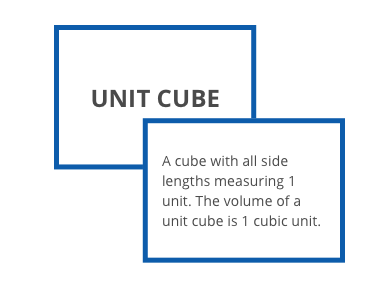# Exponents and Scientific Notation

Students learn to simplify complex-looking exponential expressions, and they learn efficient ways to describe, communicate, and operate with very large and very small numbers.

## Unit Summary

In Unit 1, eighth grade students learn how complex-looking expressions and very large or small numbers can be represented in simpler ways. Through investigation, students discover ways to write equivalent exponential expressions, and then formalize their understanding of these strategies into properties of exponents. Later in the unit, they learn efficient ways to describe, communicate, and operate with very large and very small numbers. Though there are many procedural elements in this unit, underneath these procedures are strong conceptual understandings. Throughout the unit, students look for structures and patterns that exist in exponential terms and powers of ten, and use those structures and patterns to make generalizations (MP.7 and MP.8).

In sixth grade, students wrote and evaluated expressions with exponents using the order of operations. They identified the parts of an expression, distinguishing a term from a factor from a coefficient. In eighth grade, students expand on these skills to go beyond just evaluation. They are presented with exponentials such as $\frac{3^{16}}{3^4}$  or $(x^2y)^5$ and are asked to simplify them or represent them in equivalent ways. In this way, students hone their abilities to manipulate algebraic expressions, which they will continue to do in future units in eighth grade. In fourth and fifth grades, students investigated patterns in powers of ten and how those patterns related to place value. In this unit, students will access these prior concepts and use them in representing and working with very large and small numbers.

In high school, students will need a strong understanding of exponents and exponent properties. They will apply the properties of exponents to exponential equations in order to reveal new understandings of the relationship. They will work with fractional exponents and discover the properties of rational exponents and rational numbers. In general, students’ ability to see the structure in an expression will support them in manipulating quadratic functions, operating with polynomials, and making connections between various relationships.

Pacing: 19 instructional days (15 lessons, 3 flex days, 1 assessment day)

## Assessment

This assessment accompanies Unit 1 and should be given on the suggested assessment day or after completing the unit.

### Fishtank Plus

#### Expanded Assessment Package

Learn how to use these tools with our Guide to Assessments

## Unit Prep

### Essential Understandings

?

• The properties of exponents summarize the structure and patterns that are inherent in exponential expressions. They are rooted in the conceptual meaning of exponents and provide a summary of how to efficiently work with exponential expressions.
• Scientific notation is a useful tool to conceptualize, communicate about, and operate with very large and very small numbers.
• The properties of exponents can be applied to numbers written in scientific notation to support efficient computation and comparison.
• Looking for structure and patterns in expressions can provide insight into how to manipulate and work with complex expressions, as well as lead to generalizations and properties.

### Vocabulary

?

exponential expression

base

exponent

power

scientific notation

properties of exponents

standard form/decimal form

### Fishtank Plus

#### Vocabulary Package

Additional vocabulary tools that help reinforce and support student vocabulary development.### Unit Materials, Representations and Tools

?

• scientific calculators

### Intellectual Prep

?

#### Internalization of Standards via the Unit Assessment

• Take unit assessment. Annotate for:
• Standards that align to each question
• Strategies and representations used in daily lessons
• Relationship to Essential Understandings of unit
• Lesson(s) to which the assessment points

#### Internalization of Trajectory of Unit

• Read and annotate "Unit Summary."
• Notice the progression of concepts through the unit using "Unit at a Glance"
• Essential understandings
• Connection to assessment questions
• Identify key opportunities to engage students in academic discourse. Read through our Guide to Academic Discourse and refer back to it throughout the unit.

## Common Core Standards

Key: Major Cluster Supporting Cluster Additional Cluster

### Core Standards

?

##### Expressions and Equations
• 8.EE.A.1 — Know and apply the properties of integer exponents to generate equivalent numerical expressions. For example, 3² × 3-5 = 3-3 = 1/3³ = 1/27.

• 8.EE.A.3 — Use numbers expressed in the form of a single digit times an integer power of 10 to estimate very large or very small quantities, and to express how many times as much one is than the other. For example, estimate the population of the United States as 3 × 108 and the population of the world as 7 × 109, and determine that the world population is more than 20 times larger.

• 8.EE.A.4 — Perform operations with numbers expressed in scientific notation, including problems where both decimal and scientific notation are used. Use scientific notation and choose units of appropriate size for measurements of very large or very small quantities (e.g., use millimeters per year for seafloor spreading). Interpret scientific notation that has been generated by technology.

?

• 6.EE.A.1

• 6.EE.A.2

• 6.EE.A.2.C

• 4.NBT.A.1

• 5.NBT.A.1

• 5.NBT.A.2

• 7.NS.A.2

?

• A.APR.D.6

• A.APR.D.7

• N.RN.A.1

• N.RN.A.2

• A.SSE.A.2

• A.SSE.B.3.C

### Standards for Mathematical Practice

• CCSS.MATH.PRACTICE.MP1 — Make sense of problems and persevere in solving them.

• CCSS.MATH.PRACTICE.MP2 — Reason abstractly and quantitatively.

• CCSS.MATH.PRACTICE.MP3 — Construct viable arguments and critique the reasoning of others.

• CCSS.MATH.PRACTICE.MP4 — Model with mathematics.

• CCSS.MATH.PRACTICE.MP5 — Use appropriate tools strategically.

• CCSS.MATH.PRACTICE.MP6 — Attend to precision.

• CCSS.MATH.PRACTICE.MP7 — Look for and make use of structure.

• CCSS.MATH.PRACTICE.MP8 — Look for and express regularity in repeated reasoning.